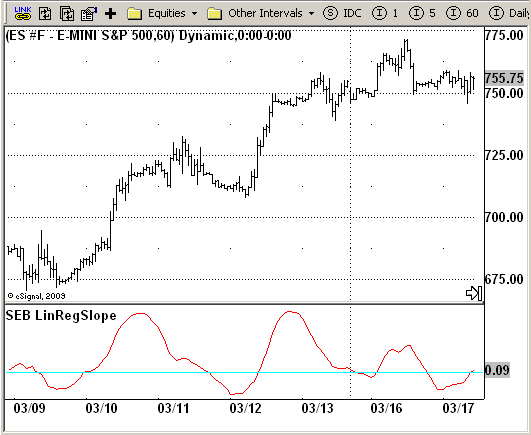# SEB Linear Regression Slope

ICE Data Services -

SEB_LinRegSlope.efs
EFSLibrary - Discussion Board

File Name: SEB_LinRegSlope.efs

Description:
This indicator plots the slope of linear regression in osccilator-like manner. This is a part of Standard Error Bands study.

Formula Parameters:

• Length : 21

Notes:
Linear Regression is a concept also know as the "least squares method" or "best fit". Linear Regression attempts to fit a straight line between several data points in such a way that distance between each data point and the line is minimized.
"Standard Error Bands" Jon Anderson, Stocks&Commodities Magazine, Traders Tips, 09/1996

SEB_LinRegSlope.efsEFS Code:

```/*********************************
Provided By:
eSignal (Copyright c eSignal), a division of Interactive Data
Formula Script (EFS) is for educational purposes only and may be
modified and saved under a new file name.  eSignal is not responsible
for the functionality once modified.  eSignal reserves the right
to modify and overwrite this EFS file with each new release.

Description:
This indicator plots the slope of linear regression in osccilator-like
manner. This is a part of Standard Error Bands study.

Version:            1.0  03/19/2009

Formula Parameters:                     Default:
Length                              21

Notes:
Linear Regression is a concept also know as the "least squares method" or
"best fit". Linear Regression attempts to fit a straight line between several
data points in such a way that distance between each data point and the line is
minimized.
"Standard Error Bands" Jon Anderson, Stocks&Commodities Magazine, Traders Tips, 09/1996
**********************************/

var fpArray = new Array();
var bInit = false;

function preMain() {
setStudyTitle("SEB LinRegSlope");
setCursorLabelName("Slope", 0);
setDefaultBarFgColor(Color.red, 0);

var x=0;
fpArray[x] = new FunctionParameter("Length", FunctionParameter.NUMBER);
with(fpArray[x++]){
setLowerLimit(1);
setDefault(21);
}
}

var xSL = null;

function main(Length) {
var nBarState = getBarState();
var nSL = 0;
if (nBarState == BARSTATE_ALLBARS) {
if (Length == null) Length = 21;
}
if (bInit == false) {
xSL = efsInternal("calc", Length);
bInit = true;
}
nSL = xSL.getValue(0);
if (nSL == null) return;
return nSL;
}

var xClose = null;
var xInit = false;

function calc(Length) {
var SL = 0;
var SumBars = Length * (Length - 1) * 0.5;
var SumSqrBars = (Length - 1) * Length * (2 * Length - 1) / 6;
var Sum1 = 0;
var SumY = 0;
var i = 0;

if(xInit==false){
xClose = close();
xInit = true;
}
for (i = 0; i < Length; i++) {
Sum1 += i * xClose.getValue(-i);
SumY += xClose.getValue(-i);
}
var Sum2 = SumBars * SumY;
var Num1 = Length * Sum1 - Sum2;
var Num2 = SumBars * SumBars - Length * SumSqrBars;
SL = Num1 / Num2;
return SL;
}```